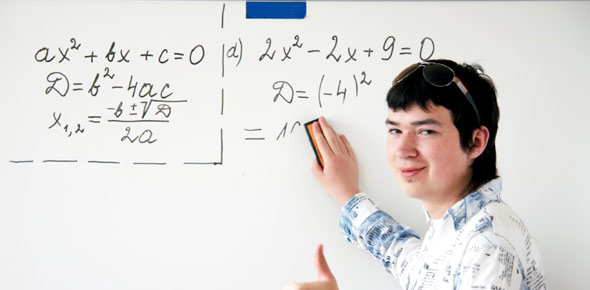3 Questions | Total Attempts: 3061SettingsUse the quadratic equation (look it up online if you cannot remember) to answer the following problems. Round your answer to the nearest tenth. Good luck!

Related Topics
• 1.
X2+ 10x + 9=0
• A.

-4.6, -9.2

• B.

-1, -3

• C.

-4.2, 6

• D.

-1, -9

• E.

7.9, -4

• 2.
2x2+ 10x + 8=0
• A.

-.5, 2

• B.

3, 20

• C.

4, 9

• D.

-2.3, -7

• E.

-1, -4

• 3.
2x2+ 4x + 2=0
• A.

-5

• B.

-1

• C.

5

• D.

40

• E.

9